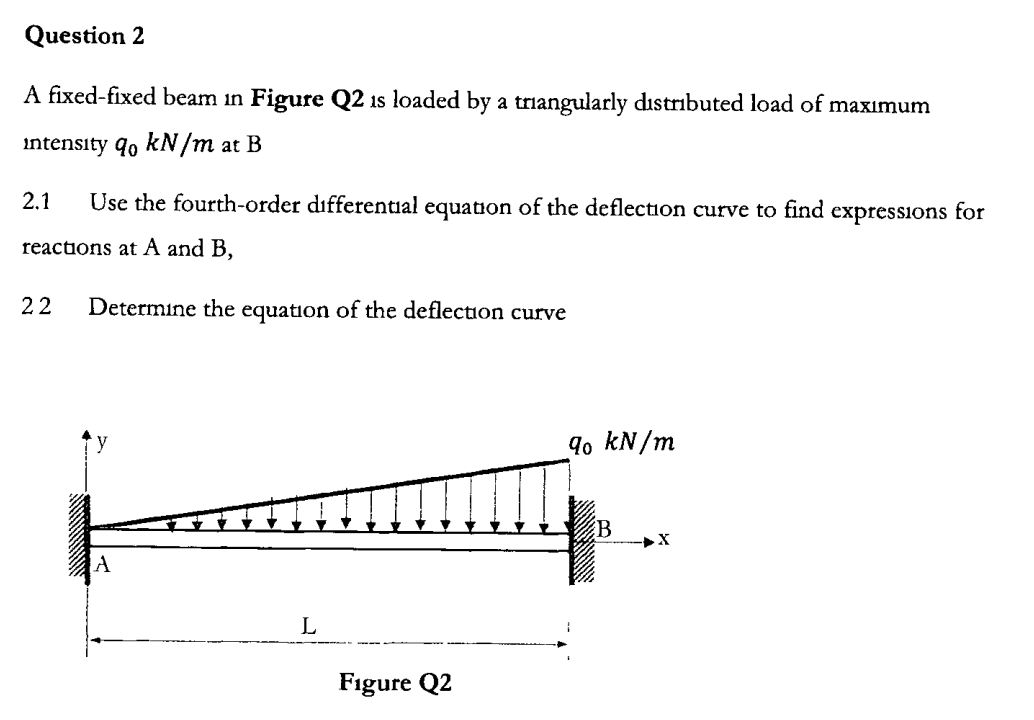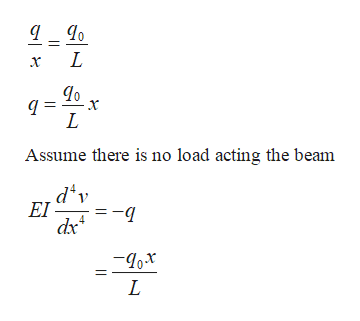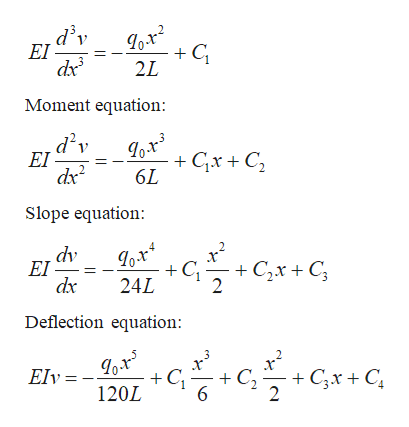Question 2A fixed-fixed beam in Figure Q2 1s loaded by a triangularly dıstributed load of maxımumintensity qo kN/m at BUse the fourth-order dıfferential equation of the deflection curve to find expressions for2.1reactions at A and B,22Determine the equatıon of the deflectuon curveo kN/myXLFigure Q2

Questionhelp_outlineImage TranscriptioncloseQuestion 2 A fixed-fixed beam in Figure Q2 1s loaded by a triangularly dıstributed load of maxımum intensity qo kN/m at B Use the fourth-order dıfferential equation of the deflection curve to find expressions for 2.1 reactions at A and B, 22 Determine the equatıon of the deflectuon curve o kN/m y X L Figure Q2 fullscreen
Step 1

Draw the free body diagram of the beam as shown in figure.

Step 2

Calculate the magnitude of load at arbitrary section.help_outlineImage TranscriptioncloseL x L Assume there is no load acting the beam d'v EI L fullscreen
Step 3

Calculate the successive integ...help_outlineImage TranscriptioncloseLo-r2 2L EI dx Moment equation: Lo-r3 GxC2 .d'v EI 6L Slope equation: dv EI dx C C2xC 24L 2 Deflection equation: +C 120L C2 Elv = CxC 6 2 fullscreen

Want to see the full answer?

See Solution

Want to see this answer and more?

Our solutions are written by experts, many with advanced degrees, and available 24/7

See Solution
Tagged in

Solid Mechanics